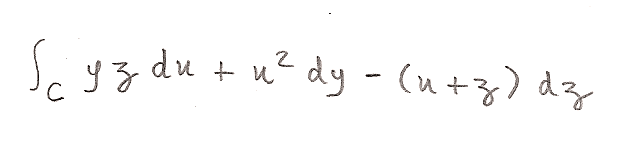# Line Integral

Consider that the curve C (IR3) is the intersection between x2+2z2=2 and y=1.

Calculate the line integral:Note: The curve C is traversed one time in the counter-clockwise direction (seen from the origin of IR3).

My attempt:

http://i.imgur.com/5EsJO.png

Can someone check? Thanks! :)

Last edited by a moderator:

haruspex
Homework Helper
Gold Member
2020 Award
If I have the question right, a lot of simplification is possible.
Given y = 1, the integral wrt y is zero, and y can be set to 1 elsewhere.
I also notice there's integral x.dz - integral z.dx. Hmmm... what do you think that might simplify to? Think of the geometric meaning, or of integration by parts.

But it is still correct?

haruspex
Homework Helper
Gold Member
2020 Award
The answer looks right. I haven't verified all the working (because it can all be done in couple of lines).

Thanks! Btw, I understand your simplifications, but I have to do this way... ;)

HallsofIvy
Homework Helper
You have curve C defined in an xyz- coordinate system but you have u, y, and z in your integral. Did you intend
$$\int_C yzdx+ x^2dy- (x+ z)dz$$?

Assuming that, since y= 1, a constant, dy= 0 so we can ignore the middle term.

I would be inclined to parameterize the curve by $x= \sqrt{2}cos(\theta)$, $y= 1$, $z= sin(\theta)$.

Then $dx= -\sqrt{2}sin(\theta)d\theta$ and $dz= cos(\theta)d\theta$.

The integral becomes
$$\int_0^{2\pi} sin(\theta)(-\sqrt{2}sin(\theta)d\theta)- (\sqrt{2}cos(\theta)+ sin(\theta))cos(\theta)d\theta$$
$$= \int_0^{2\pi}[-\sqrt{2}(sin^2(\theta)+ cos^2(\theta))- \sqrt{2}sin(\theta)cos(\theta)]d\theta$$
$$= -\sqrt{2}\int_0^{2\pi}[1+ sin(\theta)cos(\theta)]d\theta$$

The answer you give, t/2- (1/4)sin(2t), can't possibly be correct because it is a function of t and there was not even a "t" in the problem! Did you intend to evaluate that at t= 0 and $2\pi$?

Last edited by a moderator:
haruspex
Homework Helper
Gold Member
2020 Award
The answer you give, t/2- (1/4)sin(2t), can't possibly be correct because it is a function of t and there was not even a "t" in the problem! Did you intend to evaluate that at t= 0 and $2\pi$?

The way I read it, the equations involving t are explanatory notes. The answer it gives earlier is "= 0".

HallsofIvy
Homework Helper
Thank you. I had to keep scrolling from side to side!

HallsofIvy
Homework Helper
I've gone back and edited my first response, replacing the incorrect "2"s with $\sqrt{2}$. However, I still do not get 0 as the integral.

Last edited by a moderator:
I found my mistake. I wrote:
The integral becomes
$$\int_0^{2\pi} sin(\theta)(-\sqr{2}sin(\theta)d\theta)+ (\sqr{2}cos(\theta)+ sin(\theta))cos(\theta)d\theta$$
[tex]=...

It's not 0, you're right. Thanks a lot! ;)

haruspex
And I've found mine.
$\int_C zdx- (x+ z)dz = \int_C (zdx-xdz) + zdz$
$\int_C zdx$ and $\int_C xdz$ are each the area inside the loop. So I thought these cancelled too. But I overlooked that one will effectively run the opposite direction around the loop, so will add, not cancel. So the whole reduces to twice the area of the ellipse.